# 利用d3.js力导布局绘制资源拓扑图实例教程d3.js 力导向图

d3.js 是著名的数据可视化基础工具，他提供了基本的将数据映射至网页元素的能力，同时封装了大量实用的数据操作函数与图形算法。其中力导向图（Force-Directed Graph）是 d3.js 提供的一种十分经典的绘图算法。通过在二维空间里配置节点和连线，在各种各样力的作用下，节点间相互碰撞和运动并在这个过程中不断地降低能量，最终达到一种能量很低的安定状态，形成一种稳定的力导向图。

d3.js 力导向图中默认提供了 5 种作用力（以最新的 5.x 为准）：ok说干就干

``````
// 所有代码基于 typescript，省略部分代码

type INode = d3.SimulationNodeDatum & {
id: string
label: string;
isAppNode?: boolean;
};

strength: number;
};

const nodes: INode[] = [...];

const container = d3.select('container');

const svg = container.select('svg')
.attr('width', width)
.attr('height', height);

const html = container.append('div')
.attr('class', styles.HtmlContainer);

// 创建一个弹簧力，根据 link 的 strength 值决定强度
.id(node => node.id)
// 资源节点与信息节点间的 strength 小一点，信息节点间的 strength 大一点

// 在 y轴 方向上施加一个力把整个图形压扁一点
.force('yt', d3.forceY().strength(() => 0.025))
.force('yb', d3.forceY(height).strength(() => 0.025))
// 节点间相互排斥的电磁力
.force('charge', d3.forceManyBody<INode>().strength(-400))
// 避免节点相互覆盖
.force('center', d3.forceCenter(width / 2, height / 2))
.stop();

// 手动调用 tick 使布局达到稳定状态
for (let i = 0, n = Math.ceil(Math.log(simulation.alphaMin()) / Math.log(1 - simulation.alphaDecay())); i < n; ++i) {
simulation.tick();
}

const nodeElements = svg.append('g')
.selectAll('circle')
.data(nodes)
.enter().append('circle')
.attr('r', 10)
.attr('fill', getNodeColor);

const labelElements = svg.append('g')
.selectAll('text')
.data(nodes)
.enter().append('text')
.text(node => node.label)
.attr('font-size', 15);

const pathElements = svg.append('g')
.selectAll('line')
.enter().append('line')
.attr('stroke-width', 1)
.attr('stroke', '#E5E5E5');

const render = () => {
nodeElements
.attr('cx', node => node.x!)
.attr('cy', node => node.y!);
labelElements
.attr('x', node => node.x!)
.attr('y', node => node.y!);
pathElements
}

render(); ``````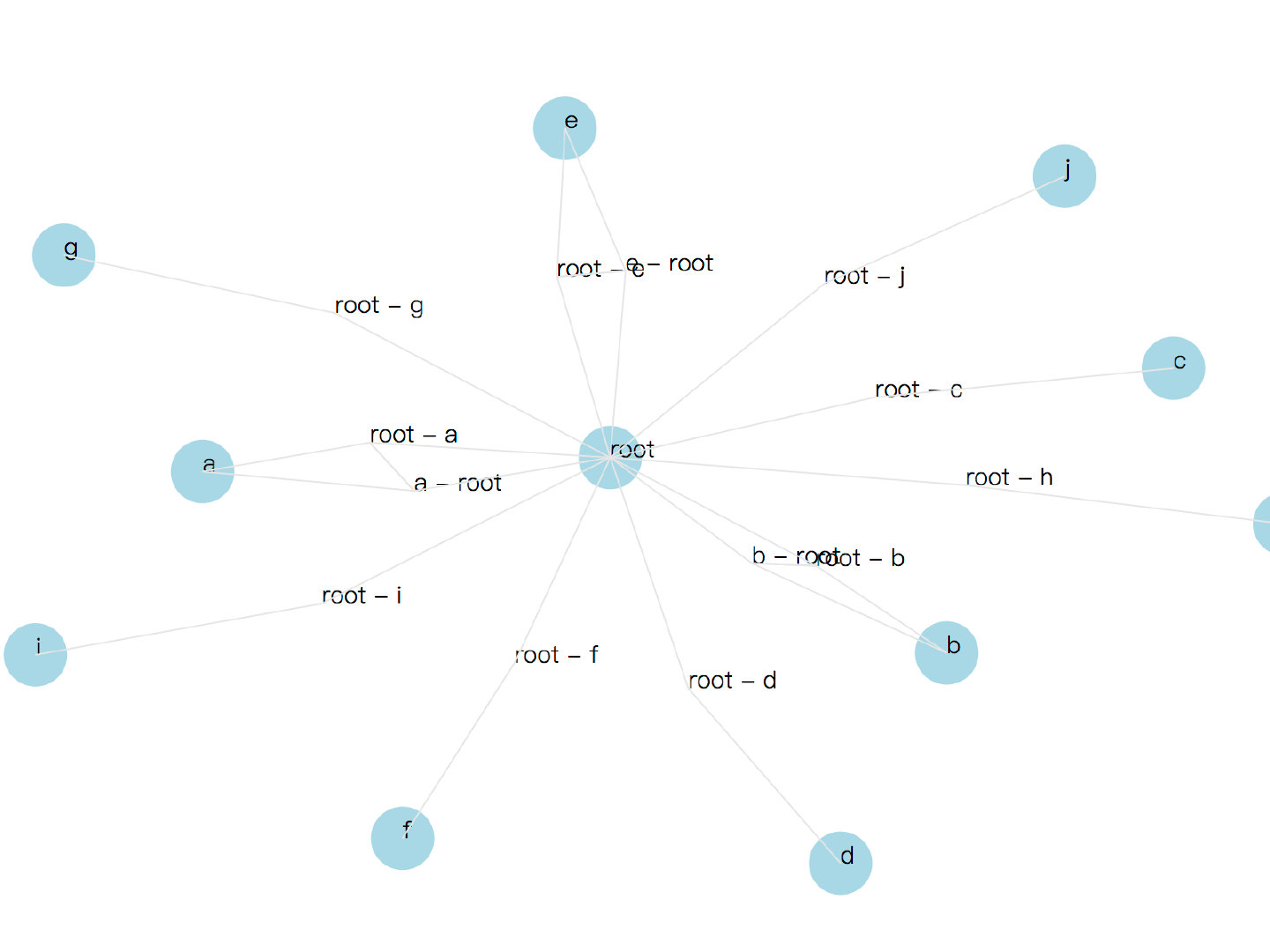ok 已经基本实现啦，那就这样啦，等后台同学实现一下接口就可以上线啦，日均UV两位数的产品要啥自行车，有的看就不错了（手动二哈）。

``````
...

const nodeElements = html.append('div')
.selectAll('div')
.data(nodes.filter(node => node.isAppNode))
.enter().append('div')
// css modules
.attr('class', styles.NodeItem)
.html((node: INode) => {
return `<p>\${node.id}</p>`;
});

const labelElements = html.append('div')
.selectAll('div')
.data(nodes.filter(node => !node.isAppNode))
.enter().append('div')
// css modules
.attr('class', styles.LabelItem)
.html(node => `
<p>\${node.label}</p>
`);

...

const render = () => {
nodeElements
.attr('style', (node) => {
return `transform: translate3d(calc(\${node.x}px - 50%), calc(\${node.y}px - 50%), 0);`;
});

labelElements
.attr('style', (node) => {
return `transform: translate3d(calc(\${node.x}px - 50%), calc(\${node.y}px - 50%), 0);`;
});
}``````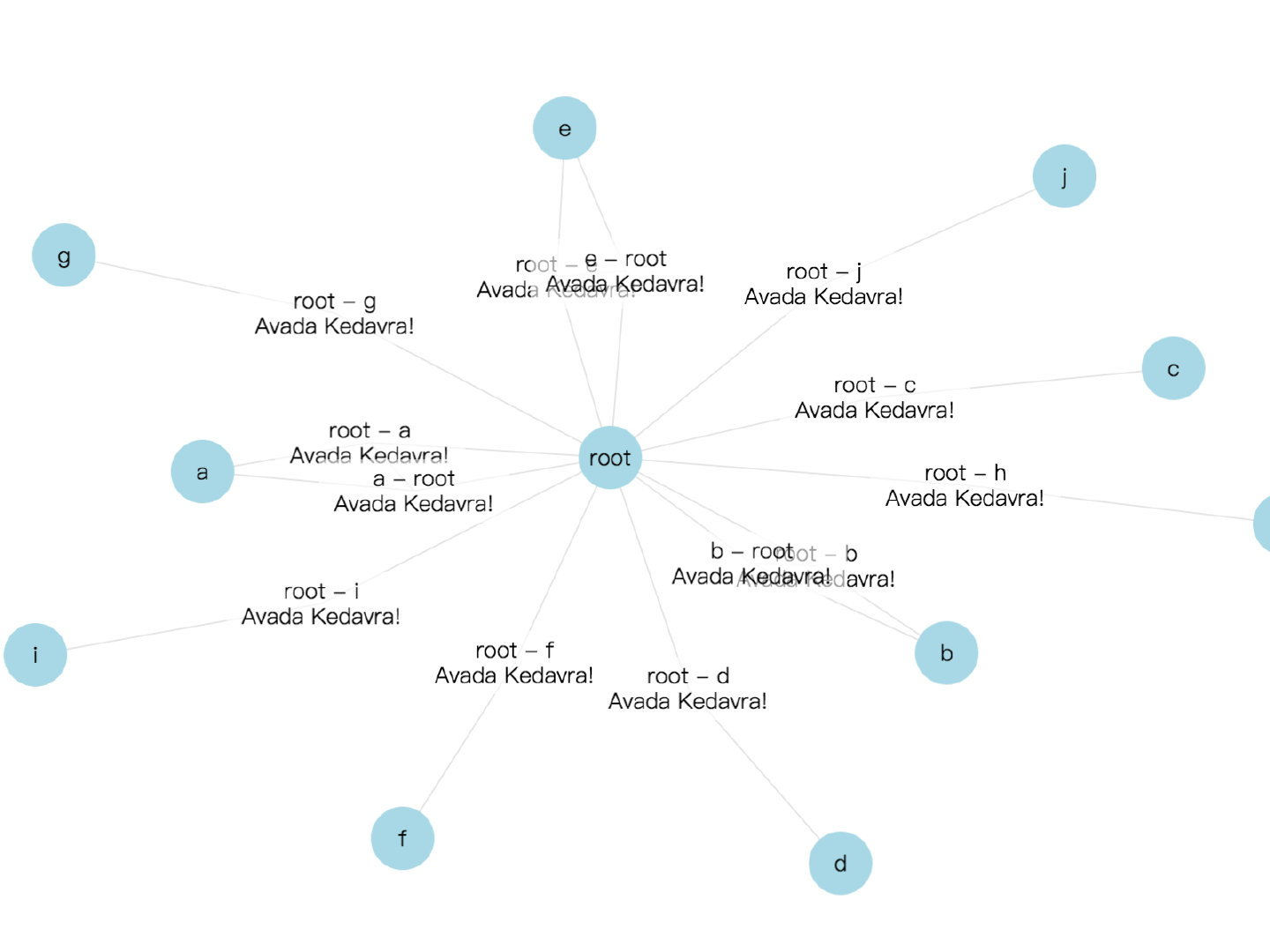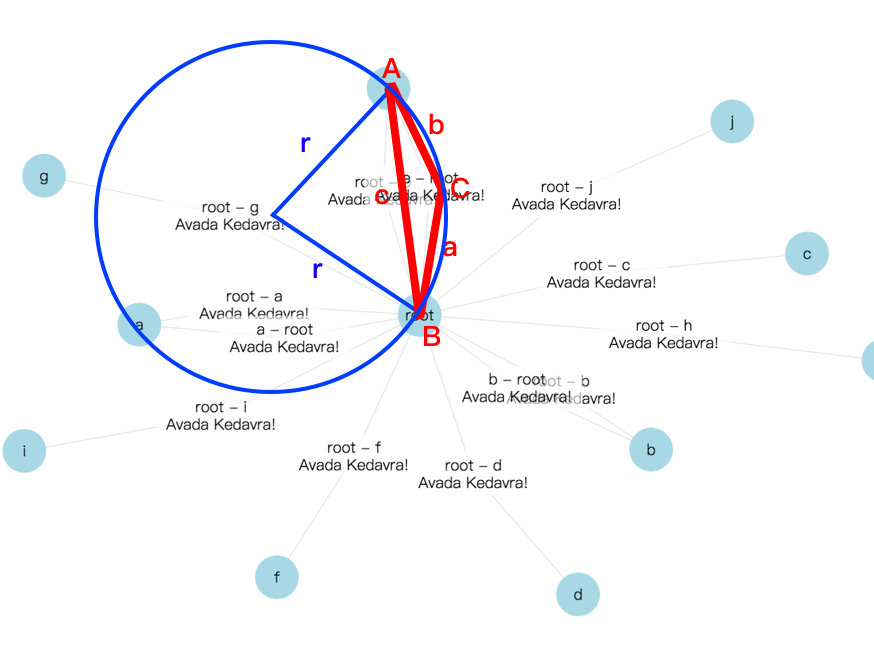``````
id: string;
start: number[];
middle: number[];
end: number[];
arcPath: string;
};

function dist(a: number[], b: number[]) {
return Math.sqrt(
Math.pow(a - b, 2) +
Math.pow(a - b, 2));
}

...

const pathElements = svg.append('g')
.selectAll('path')
.enter().append('path')
.attr('fill', 'none')
.attr('stroke-width', 1)
.attr('stroke', '#E5E5E5');

...

const render = () => {
...

nodes
// 过滤出所有的信息节点
.filter(node => !node.isAppNode)
.forEach((node) => {
...

const a = dist(B, C);
const b = dist(C, A);
const c = dist(A, B);

// 余弦定理求得∠C
const angle = Math.acos((a * a + b * b - c * c) / (2 * a * b));
// 正弦定理求得外接圆半径
const r = _.round(c / Math.sin(angle) / 2, 4);

// 角度大小flag，因为我们要的是条弧线而不是一个残缺的圆，所以恒为0
const laf = 0;

// 弧线方向flag，根据AB的斜率判断C在AB线的那一边，再确定弧线方向
const saf = +((B - A) * (C - A) - (B - A) * (C - A) < 0);

const arcPath = ['M', A, 'A', r, r, 0, laf, saf, B].join(' ');

});

pathElements
});
}``````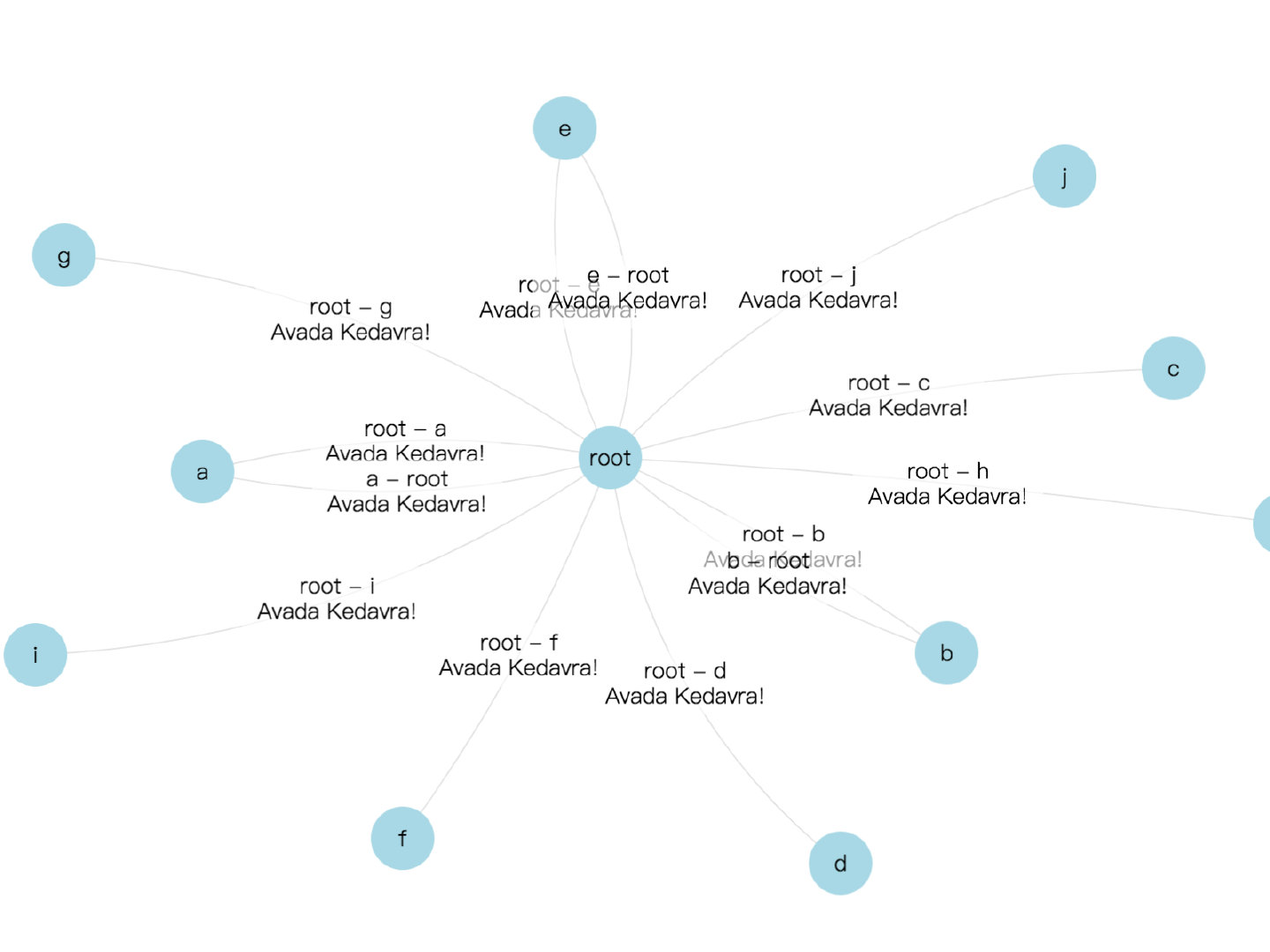``````
// 在 svg 元素中添加一个 marker 元素
<svg>
<marker
id="arrow"
viewBox="-10 -10 20 20"
markerWidth="20"
markerHeight="20"
orient="auto"
>
<path
d="M-6.75,-6.75 L 0,0 L -6.75,6.75"
fill="none"
stroke="#E5E5E5"
/>
</marker>
</svg>

...

const pathElements = svg.append('g')
.selectAll('path')
.enter().append('path')
.attr('fill', 'none')
// 设置 marker-end 属性
.attr('marker-end', 'url(#arrow)')
.attr('stroke-width', 1)
.attr('stroke', '#E5E5E5');

...``````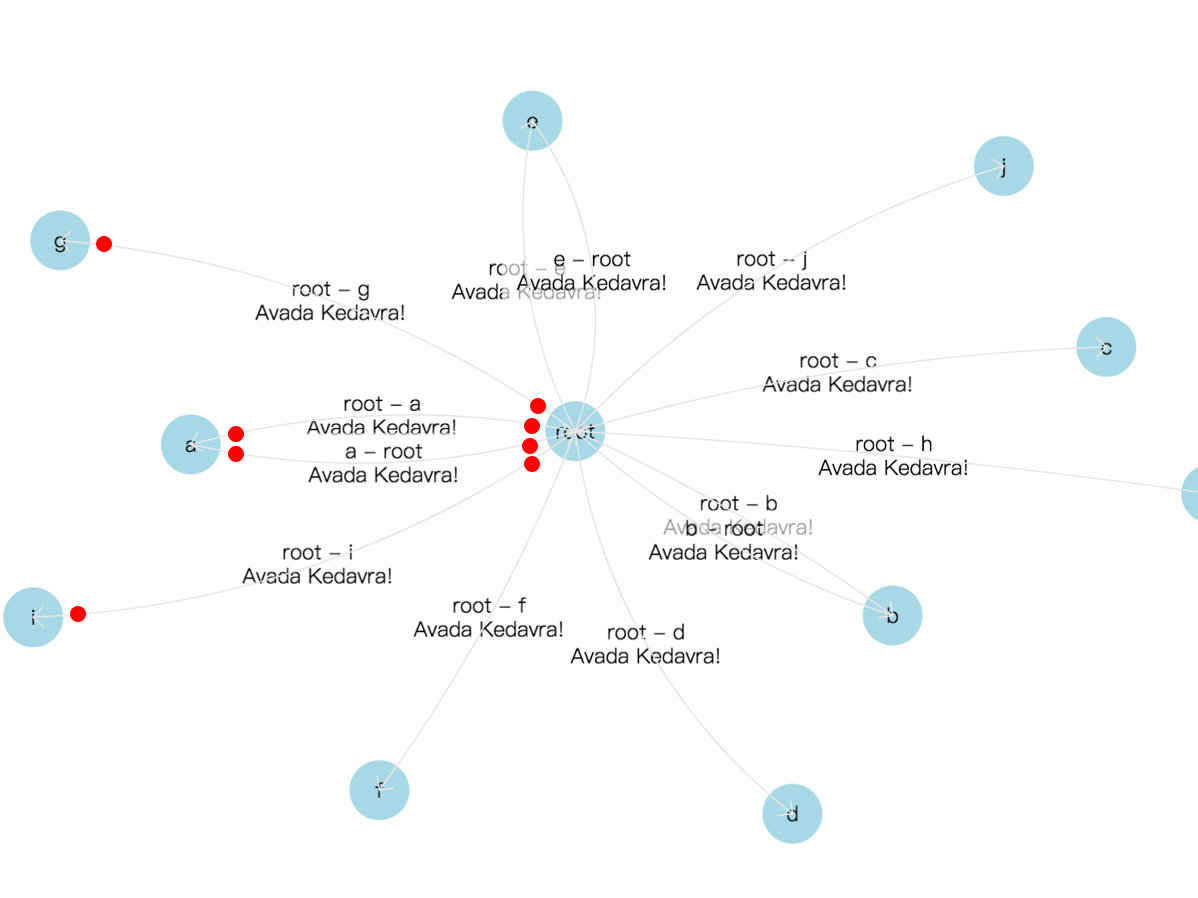``````
import intersect from 'path-intersection';

const render = () => {
...

nodes
// 过滤出所有的信息节点
.filter(node => !node.isAppNode)
.forEach((node) => {
...

const a = dist(B, C);
const b = dist(C, A);
const c = dist(A, B);

// 余弦定理求得∠C
const angle = Math.acos((a * a + b * b - c * c) / (2 * a * b));
// 正弦定理求得外接圆半径
const r = _.round(c / Math.sin(angle) / 2, 4);

// 角度大小flag，因为我们要的是条弧线而不是一个残缺的圆，所以恒为0
const laf = 0;

// 弧线方向flag，根据AB的斜率判断C在AB线的那一边，再确定弧线方向
const saf = +((B - A) * (C - A) - (B - A) * (C - A) < 0);

const origArcPath = ['M', A, 'A', r, r, 0, laf, saf, B].join(' ');

const startCirclePath = [
'M', A,
'm', [-raidus, 0],
'a', raidus, raidus, 0, 1, 0, [raidus * 2, 0],
'a', raidus, raidus, 0, 1, 0, [-raidus * 2, 0],
].join(' ');
const endCirclePath = [
'M', B,
'm', [-raidus, 0],
'a', raidus, raidus, 0, 1, 0, [raidus * 2, 0],
'a', raidus, raidus, 0, 1, 0, [-raidus * 2, 0],
].join(' ');

const startIntersection = intersect(origArcPath, startCirclePath);
const endIntersection = intersect(origArcPath, endCirclePath);

const arcPath = [
'M', [startIntersection.x, startIntersection.y],
'A', r, r, 0, laf, saf, [endIntersection.x, endIntersection.y],
].join(' ');

});

pathElements
});

...
}``````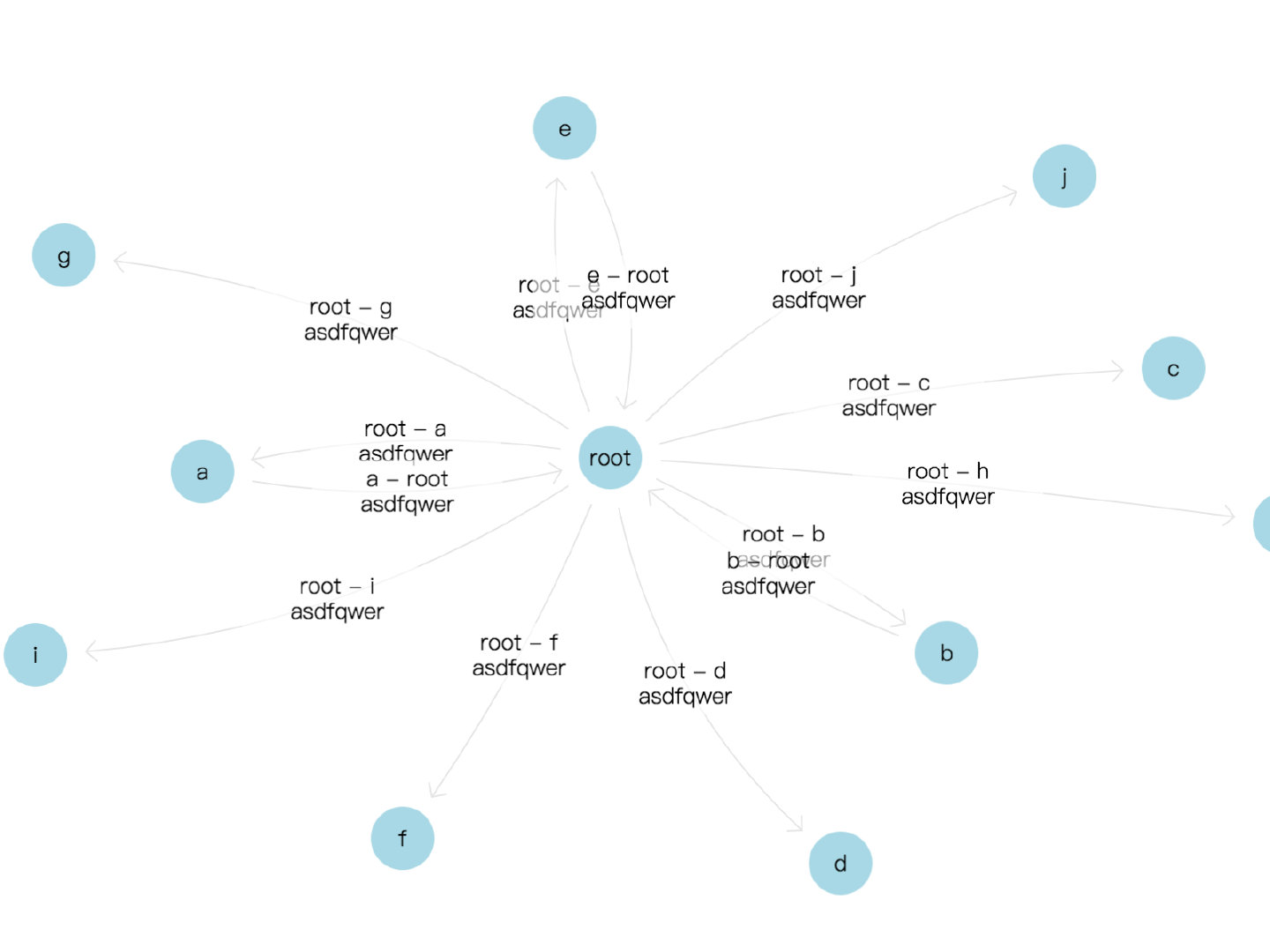``````
import { svgPathProperties } from 'svg-path-properties';

...

render = () => {
...

labelElements
const totalLength = properties.getTotalLength();
const point = properties.getPointAtLength(
);

return `transform: translate3d(calc(\${point.x}px - 50%), calc(\${point.y}px - 50%), 0);`;
});

...
}``````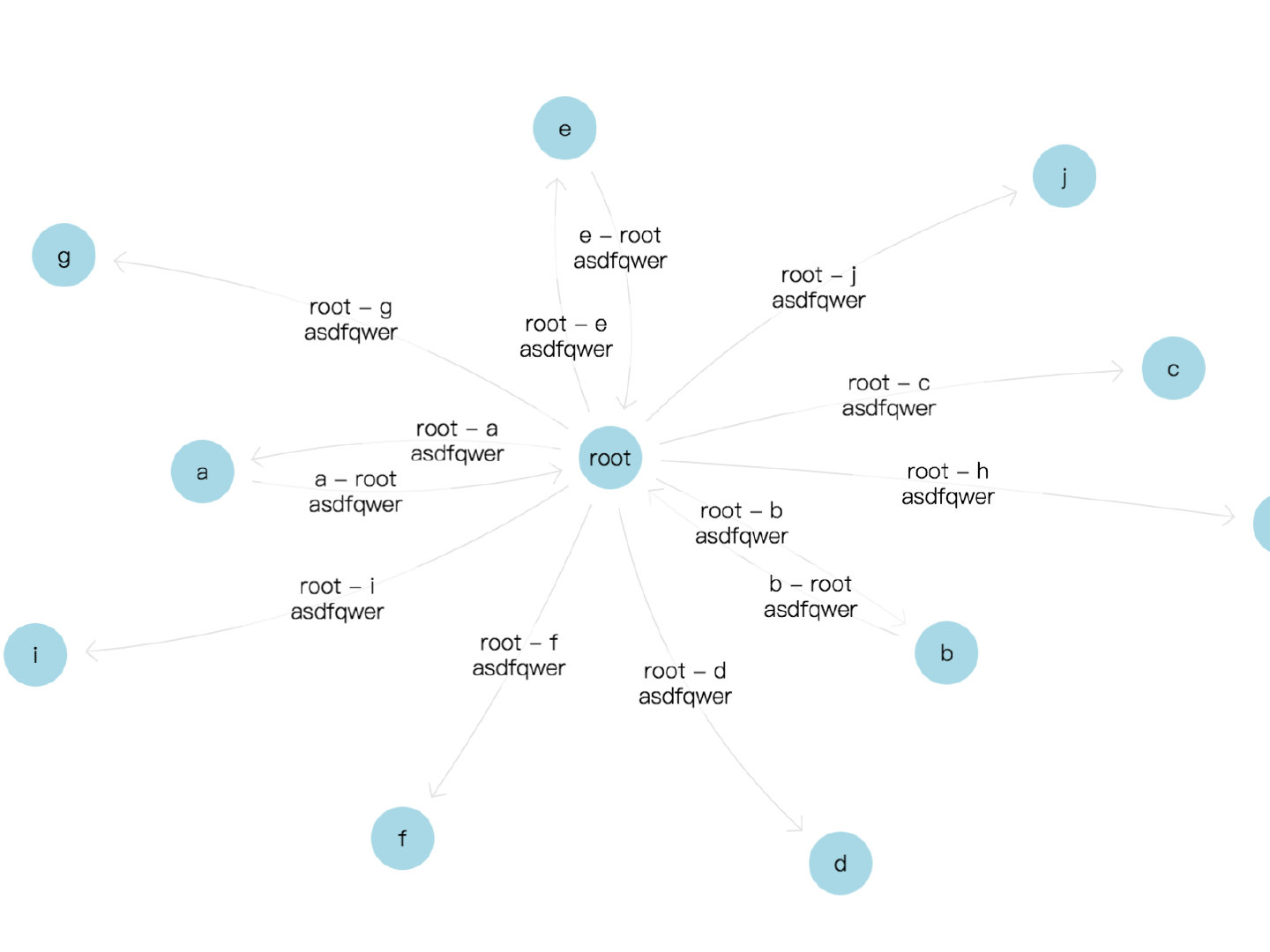• 各种力的系数，在数据不同时不能通用，还必须根据数据不同试出来一个相对通用的系数函数。
• 不能保证所有的节点都在方框内且不重叠

d3.js 拓扑图 d3.js 教程 js 绘制拓扑图